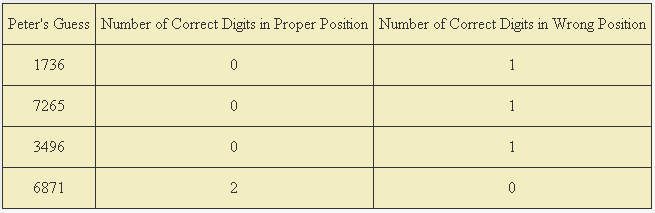# Number Game Theory

Logic Level 4

Jonathan and Peter are playing the Number Game. It is a game where one of the players try to guess a four-digit number that is secretly written by a "number dealer" in the least number of guesses possible. There are a few rules to consider though.

• No two digits can be equal.
• None of the digits can take a zero value.
• For every turn that a player tries to guess the four digit number, the number dealer will give the player two numbers. The first number gives the number of correct digits that are in the correct position, while the second number gives the number of correct digits in the wrong position.
• The dealer cannot give any other information about the number (if it is odd/even, prime, perfect, etc.)

Jonathan dealt a four digit number to Peter. Peter guessed for the number four times, and obtained the following results:What is the smallest number that Jonathan could have written?

Note: This problem is original.

×

Problem Loading...

Note Loading...

Set Loading...